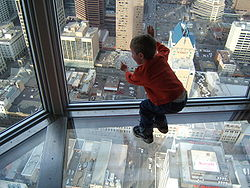# Cg Programming/Unity/Skyboxes天空盒

## 在背景渲染天空盒float4 frag(vertexOutput input) : COLOR
{
return texCUBE(_Cube, input.viewDir);
}

## 完整的着色器代码

Shader "Cg shader for skybox" {
Properties {
_Cube ("Environment Map", Cube) = "" {}
}
Tags { "Queue" = "Background" }

Pass {
ZWrite Off
Cull Front

CGPROGRAM

#pragma vertex vert
#pragma fragment frag

#include "UnityCG.cginc"

// User-specified uniforms
uniform samplerCUBE _Cube;

struct vertexInput {
float4 vertex : POSITION;
};
struct vertexOutput {
float4 pos : SV_POSITION;
float3 viewDir : TEXCOORD1;
};

vertexOutput vert(vertexInput input)
{
vertexOutput output;

float4x4 modelMatrix = _Object2World;
output.viewDir = mul(modelMatrix, input.vertex).xyz
- _WorldSpaceCameraPos;
output.pos = mul(UNITY_MATRIX_MVP, input.vertex);
return output;
}

float4 frag(vertexOutput input) : COLOR
{
return texCUBE(_Cube, input.viewDir);
}

ENDCG
}
}
}

## Unity天空盒系统的着色器代码

Shader "Cg shader for Unity-specific skybox" {
Properties {
_Cube ("Environment Map", Cube) = "white" {}
}

Tags { "Queue"="Background"  }

Pass {
ZWrite Off
Cull Off

CGPROGRAM
#pragma vertex vert
#pragma fragment frag

// User-specified uniforms
samplerCUBE _Cube;

struct vertexInput {
float4 vertex : POSITION;
float3 texcoord : TEXCOORD0;
};

struct vertexOutput {
float4 vertex : SV_POSITION;
float3 texcoord : TEXCOORD0;
};

vertexOutput vert(vertexInput input)
{
vertexOutput output;
output.vertex = mul(UNITY_MATRIX_MVP, input.vertex);
output.texcoord = input.texcoord;
return output;
}

fixed4 frag (vertexOutput input) : COLOR
{
return texCUBE (_Cube, input.texcoord);
}
ENDCG
}
}
}

## 总结

• 如何用通过方法渲染天空盒。
• 如何在Unity中不用游戏对象来渲染天空盒。

## 扩展阅读

• 关于立方体映射与对象中天空盒的反射，你应该阅读章节“反射表面”。
• 关于跟天空盒一致的光照，你应该阅读章节“多光源”。